## How to Convert Square Miles to Square Micrometer | Area Units

The image below represents square miles to square micrometers.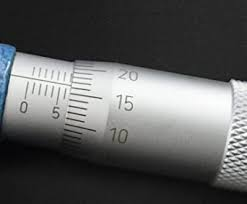To convert square miles to square micrometers, one essential parameter is needed and this parameter is Unit Value.

The formula for converting square miles to square micrometers:

1 Square Mile = 2589990000000000000 Square Micrometers

Let’s solve an example;
Find the conversion of square miles to square micrometers when the unit value is 5.

1 Square Mile = 2589990000000000000 Square Micrometers

Then,

5 Square Miles = (5 x 2589990000000000000) Square Micrometers
5 Square Miles = 12949950000000000000 Square Micrometers

Therefore, the square micrometer(s) is 12949950000000000000.

## How to Convert Acres to Square Micrometers | Area Units

The image below represents acres to square micrometers.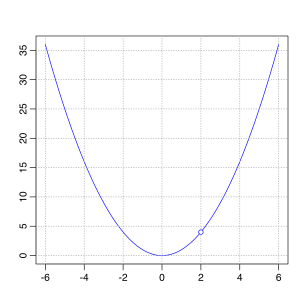To convert acres to square micrometers, one essential parameter is needed and this parameter is Unit Value.

The formula for converting acres to square micrometers:

1 Acre = 4046856422400000 Square Micrometers

Let’s solve an example;
Find the conversion of acres to square micrometers when the unit value is 2.

1 Acre = 4046856422400000 Square Micrometers

Then,

2 Acres = (2 x 4046856422400000) Square Micrometers
2 Acres = 8093712844800000 Square Micrometers

Therefore, the square micrometer(s) is 8093712844800000.

## How to Convert Square Yards to Square Micrometers | Area Units

The image below represents square yards to square micrometers.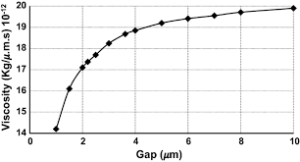To convert square yards to square micrometers, one essential parameter is needed and this parameter is Unit Value.

The formula for converting square yards to square micrometers:

1 Square Yard = 836127360000 Square Micrometers

Let’s solve an example;
Find the conversion of square yards to square micrometers when the unit value is 9.

1 Square Yard = 836127360000 Square Micrometers

9 Square Yard(s) = (9 x 836127360000) Square Micrometers
9 Square Yard(s) = 7525146240000 Square Micrometers

Therefore, the square micrometer(s) is 7525146240000.

## How to Convert Square Feet to Square Micrometers | Area Units

The image below represents square feet to square micrometers.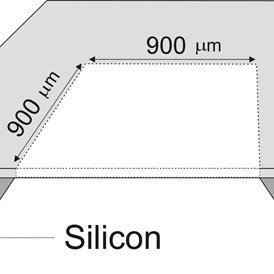To convert square feet to square micrometers, one essential parameter is needed and this parameter is Unit Value.

The formula for converting square feet to square micrometers:

1 Square Feet = 92903040000 Square Micrometers

Given an example;
Convert square feet to square micrometers when the unit value is 10.

1 Square Feet = 92903040000 Square Micrometers

Then,

10 Square Feet = (10 x 92903040000) Square Micrometers
10 Square Feet = 929030400000 Square Micrometers

Therefore, the square micrometer(s) is 929030400000.

## How to Convert Square Inches to Square Micrometers | Area Units

The image below represents square inches to square micrometers.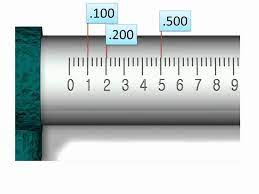To convert square inches to square micrometers, one essential parameter is needed and this parameter is Unit Value.

The formula for converting square inches to square micrometers:

1 Square Inch = 645160000 Square Micrometers

Let’s solve an example;
Convert square inches to square micrometers when the unit value is 12.

1 Square Inch = 645160000 Square Micrometers

Then,

12 Square Inches = (12 x 645160000) Square Micrometers
12 Square Inches = 7741920000 Square Micrometers

Therefore, the square micrometer(s) is 7741920000.